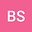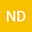Bayesian model comparison of alternative cosmologies
••• Beethoven Santos,
• N. Chandrachani Devi
Beethoven Santos
Observatório Nacional Rio de Janeiro
Author ProfileN. Chandrachani Devi
Author Profile## Abstract

In this work, we make a statistical comparison of some known cosmological models: The cosmological constant ($$\Lambda$$CDM) model, the constant equation-of-state ($$w$$CDM) model, the CPL dark energy parameterization, the Dvali-Gabadadze-Porrati (DGP) model, a vacuum-decay ($$\Lambda(t)$$CDM) model and also the power-law $$f(R)$$ model in the metric formalism. For this purpose, we perform a Bayesian model selection analysis using the Affine-Invariant Ensemble Sampler Monte-Carlo method. In order to obtain the parametric space and the posterior distribution for the parameters of each model, we use the more up-to-date type Ia supernova (SNe Ia) data, the Joint Lightcurve Analysis (JLA) compilation, containing 740 events between $$0.01<z<1.3$$. The model selection is then performed by obtaining the Bayesian evidence of each model and computing the Bayes factor between two models. The results indicate that the JLA data only cannot distinguish the standard $$\Lambda$$CDM from the $$\Lambda(t)$$CDM, power-law metric $$f(R)$$ and DGP alternatives, but to make more strong conclusions, a more robust analysis including combining the SNe Ia data with other kind of observables is necessary.